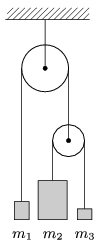Mathematical and Physical Journal
for High Schools
Issued by the MATFUND Foundation
 Already signed up? New to KöMaL?

#Problem P. 4187. (October 2009)

P. 4187. How do the objects shown in the figure move, if m1=2 kg, m2=8 kg, m3=1 kg? The mass of the pulley and the ropes is negligible.(5 pont)

Deadline expired on November 10, 2009.

Sorry, the solution is available only in Hungarian. Google translation

Megoldás. $\displaystyle a_{1}=\frac{4m_{2}m_{3}-m_{1}(m_{2}+m_{3})}{4m_{2}m_{3}+m_{1}(m_{2}+m_{3})}g=0{,}28\,g\approx 2{,}7~\frac{\rm m}{\rm s^2}$ felfelé,

$\displaystyle a_{2}=\frac{4m_{2}m_{3}+m_{1}m_{2}-3m_{1}m_{3}}{4m_{2}m_{3}+m_{1}(m_{2}+m_{3})}g=0{,}84\,g\approx 8{,}2~\frac{\rm m}{\rm s^2}$ lefelé,

$\displaystyle a_{3}=\frac{3m_{1}m_{2}-m_{1}m_{3}-4m_{2}m_{3}}{4m_{2}m_{3}+m_{1}(m_{2}+m_{3})}g=0{,}28\,g\approx 2{,}7~\frac{\rm m}{\rm s^2}$ felfelé.

### Statistics:

 183 students sent a solution. 5 points: 53 students. 4 points: 11 students. 3 points: 8 students. 2 points: 14 students. 1 point: 27 students. 0 point: 62 students. Unfair, not evaluated: 8 solutionss.

Problems in Physics of KöMaL, October 2009